# m Derived Band Stop Filter

## m Derived Band Stop Filter:

The m Derived Band Stop Filter can be derived from the prototype band elimination filter section in the exactly same way as the m-derived band pass filter.

The m Derived Band Stop Filter section is as shown in the Fig. 9.37.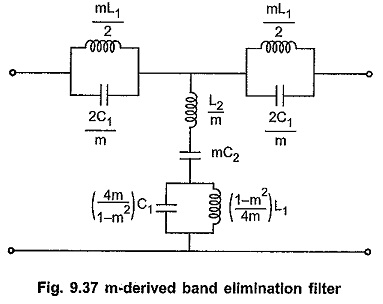The relationship between frequency of infinite attenuation (f1∞ , f2∞)  and cut-off frequencies (f1, f2) is given by,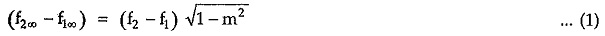If the frequency of resonance is f0 then it is the geometric mean of two cut-off frequencies as well as of two frequencies of infinite attenuation.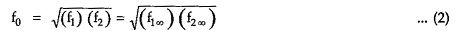Simplifying equation (1),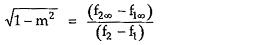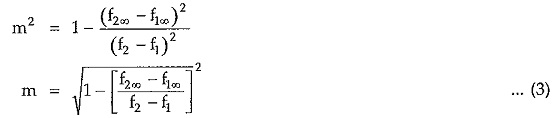#### Variations of Characteristic Impedance (Z0) , Attenuation Constant (α) and Phase Constant (β) with Frequency:

The variations of characteristic impedance Z0, attenuation constant α and phase shift β with frequency is as shown in the Fig. 9.38 (a), (b) and (c)

Updated: November 13, 2019 — 10:39 pm